# Resistor

A resistor is a two-terminal electronic component that opposes an electric current by producing a voltage drop between its terminals in proportion to the current. Resistors are used as part of electrical networks and electronic circuits.

The mathematical relationship between the electrical resistance (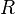$R$) of the resistor, the voltage drop ($V$) across the resistor, and the current (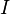$I$) flowing through the resistor is expressed by the following equation, known as Ohm's law:$V = I R$.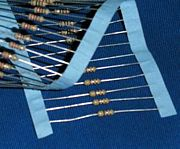Axial-lead resistors on tape. The tape is removed during assembly before the leads are formed and the part is inserted into the board.

## Identifying resistors

Most axial resistors use a pattern of colored stripes to indicate resistance. Surface-mount resistors are marked numerically. Cases are usually tan, brown, blue, or green, though other colors are occasionally found, such as dark red or dark gray.

One can also use a multimeter or ohmmeter to test the resistance values of a resistor.

### Four-band axial resistors

Four-band identification is the most commonly used color coding scheme on all resistors. It consists of four colored bands that are painted around the body of the resistor. The first two bands encode the first two significant digits of the resistance value, the third is a power-of-ten multiplier, and the fourth is the tolerance, or acceptable error, of the value.

For example, green-blue-yellow-red is 56×104 Ω = 560 kΩ ± 2 percent).

Each color corresponds to a certain number, shown in the chart below. The tolerance for a 4-band resistor will be 1 percent, 5 percent, or 10 percent.

Color 1st band 2nd band 3rd band (multiplier) 4th band (tolerance) Temp. Coefficient
Black 0 0 ×100
Brown 1 1 ×101 ±1% (F) 100 ppm
Red 2 2 ×102 ±2% (G) 50 ppm
Orange 3 3 ×103 15 ppm
Yellow 4 4 ×104 25 ppm
Green 5 5 ×105 ±0.5% (D)
Blue 6 6 ×106 ±0.25% (C)
Violet 7 7 ×107 ±0.1% (B)
Gray 8 8 ×108 ±0.05% (A)
White 9 9 ×109
Gold ×10-1 ±5% (J)
Silver ×10-2 ±10% (K)
None ±20% (M)

### Preferred values

Resistors are manufactured in values from a few milliohms to about a gigaohm; only a limited range of values from the IEC 60063 preferred number series are commonly available. These series are called E6, E12, E24, E96, and E192. The number tells how many standardized values exist in each decade (for example, between 10 and 100, or between 100 and 1000). So resistors conforming to the E12 series can have 12 distinct values between 10 and 100, whereas those confirming to the E24 series would have 24 distinct values.

In practice, the discrete component sold as a "resistor" is not a perfect resistance, as defined above. Resistors are often marked with their tolerance (maximum expected variation from the marked resistance). These E numbers correspond to the formula R = 10^(N/E), So for an 1.21 ohm E96 series resistor, N=8 and 10^(8/96)=1.21 ohm. Each multiple of 96 added to the remainder gives the next decade. So a 12.1 ohm resistor would have a N= 8+96 = 104. N can also be found by using the formula E*LOG10(R) = N.

### 5-band axial resistors

5-band identification is used for higher precision (lower tolerance) resistors (1 percent, 0.5 percent, 0.25 percent, 0.1 percent), to notate the extra digit. The first three bands represent the significant digits, the fourth is the multiplier, and the fifth is the tolerance. Five-band standard tolerance resistors are sometimes encountered, generally on older or specialized resistors. They can be identified by noting a standard tolerance color in the fourth band. The fifth band in this case is the temperature coefficient.

### SMT resistors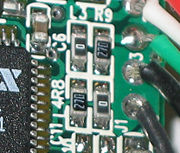This image shows four surface-mount resistors (the component at the upper left is a capacitor) including two zero-ohm resistors. Zero-ohm links are often used instead of wire links, so that they can be inserted by a resistor-inserting machine.

Surface mounted resistors are printed with numerical values in a code related to that used on axial resistors. Standard-tolerance Surface Mount Technology (SMT) resistors are marked with a three-digit code, in which the first two digits are the first two significant digits of the value and the third digit is the power of ten (the number of zeroes). For example:

 "334" = 33 × 10,000 ohms = 330 kilohms "222" = 22 × 100 ohms = 2.2 kilohms "473" = 47 × 1,000 ohms = 47 kilohms "105" = 10 × 100,000 ohms = 1 megohm

Resistances less than 100 ohms are written: 100, 220, 470. The final zero represents ten to the power zero, which is 1. For example:

 "100" = 10 × 1 ohm = 10 ohms "220" = 22 × 1 ohm = 22 ohms

Sometimes these values are marked as "10" or "22" to prevent a mistake.

Resistances less than 10 ohms have 'R' to indicate the position of the decimal point (radix point). For example:

 "4R7" = 4.7 ohms "0R22" = 0.22 ohms "0R01" = 0.01 ohms

Precision resistors are marked with a four-digit code, in which the first three digits are the significant figures and the fourth is the power of ten. For example:

 "1001" = 100 × 10 ohms = 1 kilohm "4992" = 499 × 100 ohms = 49.9 kilohm "1000" = 100 × 1 ohm = 100 ohms

"000" and "0000" sometimes appear as values on surface-mount zero-ohm links, since these have (approximately) zero resistance.

### Industrial type designation

Format: [two letters]<space>[resistance value (three digit)]<nospace>[tolerance code(numerical - one digit)] 

Power Rating at 70 °C
Type No. Power
rating
(watts)
MIL-R-11
Style
MIL-R-39008
Style
BB 1/8 RC05 RCR05
CB ¼ RC07 RCR07
EB ½ RC20 RCR20
GB 1 RC32 RCR32
HB 2 RC42 RCR42
GM 3 - -
HM 4 - -
Tolerance Code
Industrial type designation Tolerance MIL Designation
5 ±5% J
2 ±20% M
1 ±10% K
- ±2% G
- ±1% F
- ±0.5% D
- ±0.25% C
- ±0.1% B

• Commercial grade: 0 °C to 70 °C
• Industrial grade: −40 °C to 85 °C (sometimes −25 °C to 85 °C)
• Military grade: −55 °C to 125 °C (sometimes -65 °C to 275 °C)
• Standard Grade -5°C to 60°C

## Resistor standards

• MIL-R-11
• MIL-R-39008
• MIL-R-39017
• MIL-PRF-26
• MIL-PRF-39007
• MIL-PRF-55342
• MIL-PRF-914
• BS 1852
• EIA-RS-279

There are other United States military procurement MIL-R- standards.

### Power dissipation

The power dissipated by a resistor is the voltage across the resistor multiplied by the current through the resistor: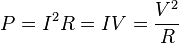$P = I^2 R = I V = \frac{V^2}{R}$

All three equations are equivalent. The first is derived from Joule's law, and the other two are derived from that by Ohm's law.

The total amount of heat energy released is the integral of the power over time: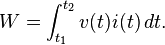$W = \int_{t_1}^{t_2} v(t) i(t)\, dt.$

If the average power dissipated exceeds the power rating of the resistor, the resistor may depart from its nominal resistance, and may be damaged by overheating. Excessive power dissipation may raise the temperature of the resistor to a point where it burns out, which could cause a fire in adjacent components and materials.

### Series and parallel circuits

When resistors are in a parallel configuration, each one has the same potential difference (voltage) across it. To find their total equivalent resistance (Req):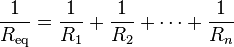$\frac{1}{R_\mathrm{eq}} = \frac{1}{R_1} + \frac{1}{R_2} + \cdots + \frac{1}{R_n}$

The parallel property can be represented in equations by two vertical lines "||" (as in geometry) to simplify equations. For two resistors,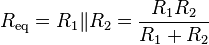$R_\mathrm{eq} = R_1 \| R_2 = {R_1 R_2 \over R_1 + R_2}$

The current through resistors in series stays the same, but the voltage across each resistor can be different. The sum of the potential differences (voltage) is equal to the total voltage. To find their total resistance: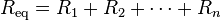$R_\mathrm{eq} = R_1 + R_2 + \cdots + R_n$

A resistor network that is a combination of parallel and series can sometimes be broken up into smaller parts that are either one or the other. For instance,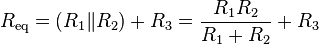$R_\mathrm{eq} = \left( R_1 \| R_2 \right) + R_3 = {R_1 R_2 \over R_1 + R_2} + R_3$

However, many resistor networks cannot be split up in this way. Consider a cube, each edge of which has been replaced by a resistor. For example, determining the resistance between two opposite vertices requires matrix methods for the general case. However, if all twelve resistors are equal, the corner-to-corner resistance is 56 of any one of them.

## Technology

### Carbon composition

Carbon composition resistors consist of a solid cylindrical resistive element with embedded wire leadouts or metal end caps to which the leadout wires are attached, which is protected with paint or plastic.

The resistive element is made from a mixture of finely ground (powdered) carbon and an insulating material (usually ceramic). The mixture is held together by a resin. The resistance is determined by the ratio of the fill material (the powdered ceramic) and the carbon. Higher concentrations of carbon, a weak conductor, result in lower resistance. Carbon composition resistors were commonly used in the 1960s and earlier, but are not so popular for general use now as other types have better specifications, such as tolerance, voltage dependence, and stress (carbon composition resistors will change value when stressed with over-voltages).

### Carbon film

A spiral is used to increase the length and decrease the width of the film, which increases the resistance. Varying shapes, coupled with the resistivity of carbon, (ranging from 9 to 40 µΩm) can make for a variety of resistances.

### Thick and thin film

Thick film resistors became popular during the 1970s, and most SMD resistors today are of this type. The principal difference between "thin film" and "thick film resistors" isn't necessarily the "thickness" of the film, but rather, how the film is applied to the cylinder (axial resistors) or the surface (SMD resistors). In thick film resistors, the "film" is applied using traditional screen-printing technology.

Thin film resistors are made by sputtering the resistive material onto the surface of the resistor. Sputtering is a method used in vacuum deposition. The thin film is then etched in a similar manner to the old (subtractive) process for making printed circuit boards: For example, the surface is coated with a photo-sensitive material, then covered by a film, irradiated with ultraviolet light, and then the exposed photo-sensitive coating, and underlying thin film, are etched away.

Thin film resistors, like their thick film counterparts, are then usually trimmed to an accurate value by abrasive or laser trimming.

Because the time during which the sputtering is performed can be controlled, the thickness of the film of a thin-film resistor can be accurately controlled. The type of the material is also usually different consisting of one or more ceramic (cermet) conductors such as tantalum nitride (TaN), ruthenium dioxide (RuO2), lead oxide (PbO), bismuth ruthenate (Bi2Ru2O7), nickel chromium (NiCr), and/or bismuth iridate (Bi2Ir2O7).

By contrast, thick film resistors, may use the same conductive ceramics, but they are mixed with sintered (powdered) glass, and some kind of liquid so that the composite can be screen-printed. This composite of glass and conductive ceramic (cermet) material is then fused (baked) in an oven at about 850 °C.

Traditionally thick film resistors had tolerances of 5 percent, but in the last few decades, standard tolerances have improved to 2 and 1 percent. But beware, temperature coefficients of thick film resistors are typically ±200 or ±250 ppm/K, depending on the resistance. Thus a 40 kelvin (70° F) temperature change can add another 1 percent variation to a 1 percent resistor.

Thin film resistors are usually specified with tolerances of 0.1, 0.2, 0.5, and 1 percent, and with temperature coefficients of 5 to 25 ppm/K. They are usually far more expensive than their thick film cousins. Note, though, that SMD thin film resistors, with 0.5 percent tolerances, and with 25 ppm/K temperature coefficients, when bought in full size reel quantities, are about twice the cost of a 1 percent, 250 ppm/K thick film resistors.

### Metal film

A common type of axial resistor today is referred to as a metal-film resistor. MELF (Metal Electrode Leadless Face) resistors often use the same technology, but are a cylindrically shaped resistor designed for surface mounting. (Note that other types of resistors, for example, carbon composition, are also available in "MELF" packages.)

Metal film resistors are usually coated with nickel chromium (NiCr), but might be coated with any of the cermet materials listed above for thin film resistors. Unlike thin film resistors, the material may be applied using different techniques than sputtering (though that is one such technique). Also, unlike thin-film resistors, the resistance value is determined by cutting a helix through the coating rather than by etching. (This is similar to the way carbon resistors are made.) The result is a reasonable tolerance (0.5, 1, or 2 percent) and a temperature coefficient of (usually) 25 or 50 ppm/K.

### Wirewound

Wirewound resistors are commonly made by winding a metal wire around a ceramic, plastic, or fiberglass core. The ends of the wire are soldered or welded to two caps, attached to the ends of the core. The assembly is protected with a layer of paint, molded plastic, or an enamel coating baked at high temperature. The wire leads are usually between 0.6 and 0.8 mm in diameter and tinned for ease of soldering. For higher power wirewound resistors, either a ceramic outer case or an aluminum outer case on top of an insulating layer is used. The aluminum cased types are designed to be attached to a heatsink to dissipate the heat; the rated power is dependent on being used with a suitable heatsink, for exmaple, a 50 W power rated resistor will overheat at around one fifth of the power dissipation if not used with a heatsink.

Because wirewound resistors are coils they have more inductance than other types of resistor, although this property can be minimized by winding the wire in sections with alternately reversed direction.

### Foil resistor

Foil resistors have had the best precision and stability ever since they were introduced in 1958, by Felix Zandman. One of the important parameters influencing stability is the temperature coefficient of resistance (TCR). Although the TCR of foil resistors is considered extremely low, this characteristic has been further refined over the years.

### Grid resistor

The term "grid resistor" can mean either of two things:

In vacuum tube or valve electronic circuit design, a grid resistor or "grid stopper" is used to limit grid current and prevent high frequencies from entering or oscillating the circuit. Such a resistor can be composed of any one of a number of resistor technologies. One application that uses grid resistors is electric guitar amplifier circuitry.

In heavy duty, industrial, high-current applications, a grid resistor is a large convection-cooled lattice of stamped metal alloy strips connected in rows between two electrodes. Such industrial grade resistors can be as large as a refrigerator; some designs can handle over 500 amperes of current, with a range of resistances extending lower than 0.04 ohm. They are used in applications such as dynamic braking for locomotives and trams, neutral grounding for industrial AC distribution, control loads for cranes and heavy equipment, load testing of generators and harmonic filtering for electric substations.

### Strain gauges

Invented by Edward E. Simmons and Arthur C. Ruge in 1938, the strain gauge consists of a resistor that changes value with applied strain. The strain resistor may be applied singly, in pairs (half bridge), or four resistors connected in a Wheatstone bridge configuration. The strain resistor is bonded with adhesive to an object that will be subjected to the strain force. With the strain gauge and a filter, amplifier, and an analog/digital converter, it is possible to directly measure the strain on an object.

### Other types

• Metal-oxide resistor
• Cermet
• Phenolic
• Tantalum
• Water resistor

## Noise

In precision circuits, electronic noise becomes of utmost concern. As dissipative elements, resistors will naturally produce a fluctuating "noise" voltage across their terminals. This Johnson–Nyquist noise is predicted by the fluctuation–dissipation theorem and is a fundamental noise source present in all resistors which must be considered in constructing low-noise electronics. For example, the gain in a simple (non-)inverting amplifier is set using a voltage divider. Noise considerations dictate that the smallest practical resistance should be used, since the noise voltage scales with resistance, and any resistor noise in the voltage divider will be impressed upon the amplifier's output.

Although Johnson–Nyquist noise is a fundamental noise source, resistors frequently exhibit other, "non-fundamental" sources of noise. Noise due to these sources is called "excess noise." Thick-film and carbon composition resistors are notorious for excess noise at low frequencies. Wire-wound and thin-film resistors, though much more expensive, are often utilized for their better noise characteristics.

## Failure modes and pitfalls

Like every part, resistors can fail; the usual way depends on their construction. Carbon composition resistors and metal film resistors typically fail as open circuits. Carbon-film resistors typically fail as short circuits. Carbon film and composition resistors can burn if too much power is dissipated. This is also possible but less likely with metal film and wirewound resistors. If not enclosed, wirewound resistors can corrode. Carbon composition resistors are prone to drifting over time and are easily damaged by excessive heat in soldering (the binder evaporates).

Various effects become important in high-precision applications. Small voltage differentials may appear on the resistors due to thermoelectric effect if their ends are not kept at the same temperature. The voltages appear in the junctions of the resistor leads with the circuit board and with the resistor body. Common metal film resistors show such effect at magnitude of about 20 µV/°C. Some carbon composition resistors can go as high as 400 µV/°C, and specially constructed resistors can go as low as 0.05 µV/°C. In applications where thermoelectric effects may become important, care has to be taken (for example) to mount the resistors horizontally to avoid temperature gradients and to mind the air flow over the board.